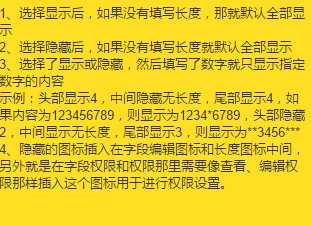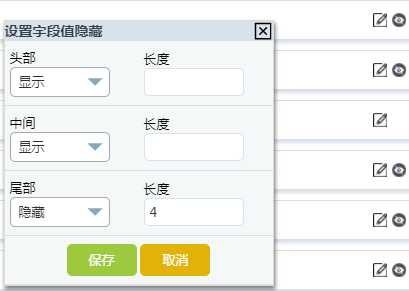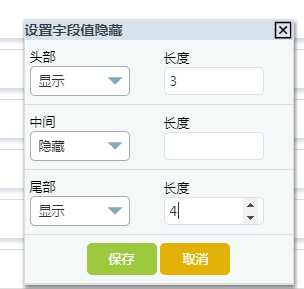tofacebook.com -专业IT技术社区(指牛堂) php实现隐藏字符串的功能 Web程序 - 指牛堂-专业IT技术平台142°

# php实现隐藏字符串的功能 Web程序

好久没有认真写自己的博客了，之前的随笔都是自己写的一部分再复制别人的代码的。因为深知自己的水平还是菜鸟级别，写不出来高质量的博客。所以自己之前写的博客都没有公开，但是万事开头难嘛，今天鼓起勇气去走这一步。工作中有个需求，就是用“*”号隐藏字符串的内容，今天准备用随笔的形式记录一下具体是怎么完成这个功能的。

（这里是用户的需求：那么我们实现的过程大致主要分为以下三个步骤：

1：实现一个界面让用户针对某个字段的值自定义隐藏的位置以及每个位置的长度。

2：将用户配置的值用分隔符保存成字符串的形式保存到数据库中。

3：将用户设定的字段的值实现具体的隐藏效果。

（注：以下具体实现的内容根据我们公司的项目为例）

一、 实现一个界面让用户针对某个字段的值自定义隐藏的位置以及每个位置的长度。

①在布局设计中，用户在当前字段点击编辑字段隐藏时，创建一个div。具体效果如下。(这个太简单，具体实现过程忽略)二、将用户配置的值用分隔符保存成字符串的形式保存到数据库中。

当用户设置好后点击保存时，js验证用户设置的值是否合理。如：全部为空。验证通过后通过ajax保存到数据库。以上图为例，我保存在表中的字符串形式是encryptflv:show_.show_.hide_4,(具体实现过程忽略)

三、将用户设定的字段的值实现具体的隐藏效果。

这是整个功能中重要的一步。

①首先是把字符串取出来，再切割为数组。

②将数组按执行隐藏的优先级进行排序```foreach (\$encryptflv as \$k => \$v) {
list(\$type, \$value) = explode(‘_‘, \$v);
if (!\$value) {
\$isnumber = ‘0‘;
\$value = ‘?‘;
} else {
\$isnumber = ‘1‘;
}
\$encrtpyflvarr[] = array(‘length‘ => \$value, ‘isnumber‘ => \$isnumber, ‘position‘ => \$k, ‘method‘ => \$type);
unset(\$k);unset(\$v);
}```

```//多维不固定参数的数组排序。
function sortArrByManyField(){
//将函数的所有参数转化为一个数组
\$args = func_get_args();
if(empty(\$args)){
return null;
}
//删除\$args的第一个元素并赋值给\$arr
\$arr = array_shift(\$args);
if(!is_array(\$arr)){
throw new Exception("第一个参数不为数组");
}
foreach(\$args as \$key => \$field){
if(is_string(\$field)){
\$temp = array();
foreach(\$arr as \$index=> \$val){
\$temp[\$index] = \$val[\$field];
}
\$args[\$key] = \$temp;
}
}
\$args[] = &\$arr;//引用值
//调用回调函数array_multisort 并将\$arhs作为回调函数的参数传入
call_user_func_array(‘array_multisort‘,\$args);
return array_pop(\$args);
}```

```//给设置的隐藏显示字数的操作转换为数组的形式，并且按照先后顺序进行排序。
\$encrtpyflvarr = sortArrByManyField(\$encrtpyflvarr, ‘isnumber‘, SORT_DESC, ‘position‘, SORT_ASC);```

③遍历这个按照多个参数排序后的多维数组，根据数组里面的长度、位置进行具体的‘*’号替换，由于代码写的比较乱（烂），这里我就不贴上来了。替换有一个函数substr_replace，但是因为我们替换的字符串有中文，那么这个函数显然不行；所以这里自己实现了一个可以替换中文字符串的函数：

```function mb_substr_replace(\$string, \$replacement, \$start, \$length = null, \$encoding = null)
{
if (extension_loaded(‘mbstring‘) === true) {
\$string_length = (is_null(\$encoding) === true) ? mb_strlen(\$string) : mb_strlen(\$string, \$encoding);
if (\$start < 0) {
\$start = max(0, \$string_length + \$start);
} else if (\$start > \$string_length) {
\$start = \$string_length;
}
if (\$length < 0) {
\$length = max(0, \$string_length - \$start + \$length);
} else if ((is_null(\$length) === true) || (\$length > \$string_length)) {
\$length = \$string_length;
}
if ((\$start + \$length) > \$string_length) {
\$length = \$string_length - \$start;
}

if (is_null(\$encoding) === true) {
return mb_substr(\$string, 0, \$start) . \$replacement . mb_substr(\$string, \$start + \$length, \$string_length - \$start - \$length);
}
return mb_substr(\$string, 0, \$start, \$encoding) . \$replacement . mb_substr(\$string, \$start + \$length, \$string_length - \$start - \$length, \$encoding);
}
return (is_null(\$length) === true) ? substr_replace(\$string, \$replacement, \$start) : substr_replace(\$string, \$replacement, \$start, \$length);
}```

总结：整个功能其实很简单，这个博客可能对于其他人来说没有什么意义。但是因为自己还是个菜鸟，主要还是为了自己记录一下吧。后面具体替换的时候还遇到个问题，<a>了开发考了多</a>。只需要替换a标签中的值，那我们用了正则匹配才完成功能。

php实现隐藏字符串的功能

我有话说: